Search by Topic

Resources tagged with Addition & subtraction similar to Crossings:

Filter by: Content type:
Age range:
Challenge level:

There are 211 results

Broad Topics > Calculations and Numerical Methods > Addition & subtractionMultiply the Addition Square

Age 11 to 14 Challenge Level:

If you take a three by three square on a 1-10 addition square and multiply the diagonally opposite numbers together, what is the difference between these products. Why?Number Tracks

Age 7 to 11 Challenge Level:

Ben’s class were cutting up number tracks. First they cut them into twos and added up the numbers on each piece. What patterns could they see?Have You Got It?

Age 11 to 14 Challenge Level:

Can you explain the strategy for winning this game with any target?A Mixed-up Clock

Age 7 to 11 Challenge Level:

There is a clock-face where the numbers have become all mixed up. Can you find out where all the numbers have got to from these ten statements?Chameleons

Age 11 to 14 Challenge Level:

Whenever two chameleons of different colours meet they change colour to the third colour. Describe the shortest sequence of meetings in which all the chameleons change to green if you start with 12. . . .Got it for Two

Age 7 to 14 Challenge Level:

Got It game for an adult and child. How can you play so that you know you will always win?As Easy as 1,2,3

Age 11 to 14 Challenge Level:

When I type a sequence of letters my calculator gives the product of all the numbers in the corresponding memories. What numbers should I store so that when I type 'ONE' it returns 1, and when I type. . . .Rabbits in the Pen

Age 7 to 11 Challenge Level:

Using the statements, can you work out how many of each type of rabbit there are in these pens?Book Codes

Age 7 to 11 Challenge Level:

Look on the back of any modern book and you will find an ISBN code. Take this code and calculate this sum in the way shown. Can you see what the answers always have in common?Neighbours

Age 7 to 11 Challenge Level:

In a square in which the houses are evenly spaced, numbers 3 and 10 are opposite each other. What is the smallest and what is the largest possible number of houses in the square?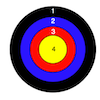Dart Target

Age 7 to 11 Challenge Level:

This task, written for the National Young Mathematicians' Award 2016, invites you to explore the different combinations of scores that you might get on these dart boards.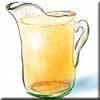Pouring the Punch Drink

Age 7 to 11 Challenge Level:

There are 4 jugs which hold 9 litres, 7 litres, 4 litres and 2 litres. Find a way to pour 9 litres of drink from one jug to another until you are left with exactly 3 litres in three of the jugs.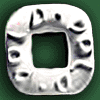Polo Square

Age 7 to 11 Challenge Level:

Arrange eight of the numbers between 1 and 9 in the Polo Square below so that each side adds to the same total.Aba

Age 11 to 14 Challenge Level:

In the following sum the letters A, B, C, D, E and F stand for six distinct digits. Find all the ways of replacing the letters with digits so that the arithmetic is correct.Tis Unique

Age 11 to 14 Challenge Level:

This addition sum uses all ten digits 0, 1, 2...9 exactly once. Find the sum and show that the one you give is the only possibility.Zargon Glasses

Age 7 to 11 Challenge Level:

Zumf makes spectacles for the residents of the planet Zargon, who have either 3 eyes or 4 eyes. How many lenses will Zumf need to make all the different orders for 9 families?Got it Article

Age 7 to 14

This article gives you a few ideas for understanding the Got It! game and how you might find a winning strategy.Like Powers

Age 11 to 14 Challenge Level:

Investigate $1^n + 19^n + 20^n + 51^n + 57^n + 80^n + 82^n$ and $2^n + 12^n + 31^n + 40^n + 69^n + 71^n + 85^n$ for different values of n.Oddly

Age 7 to 11 Challenge Level:

Find the sum of all three-digit numbers each of whose digits is odd.Age 5 to 11 Challenge Level:

Try out this number trick. What happens with different starting numbers? What do you notice?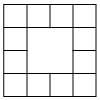Prison Cells

Age 7 to 11 Challenge Level:

There are 78 prisoners in a square cell block of twelve cells. The clever prison warder arranged them so there were 25 along each wall of the prison block. How did he do it?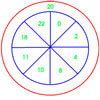On Target

Age 7 to 11 Challenge Level:

You have 5 darts and your target score is 44. How many different ways could you score 44?Jugs of Wine

Age 11 to 14 Challenge Level:

You have four jugs of 9, 7, 4 and 2 litres capacity. The 9 litre jug is full of wine, the others are empty. Can you divide the wine into three equal quantities?Zios and Zepts

Age 7 to 11 Challenge Level:

On the planet Vuv there are two sorts of creatures. The Zios have 3 legs and the Zepts have 7 legs. The great planetary explorer Nico counted 52 legs. How many Zios and how many Zepts were there?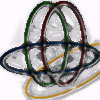Bean Bags for Bernard's Bag

Age 7 to 11 Challenge Level:

How could you put eight beanbags in the hoops so that there are four in the blue hoop, five in the red and six in the yellow? Can you find all the ways of doing this?Age 7 to 11 Challenge Level:

Can you put plus signs in so this is true? 1 2 3 4 5 6 7 8 9 = 99 How many ways can you do it?A-magical Number Maze

Age 7 to 11 Challenge Level:

This magic square has operations written in it, to make it into a maze. Start wherever you like, go through every cell and go out a total of 15!Pyramids

Age 11 to 14 Challenge Level:

What are the missing numbers in the pyramids?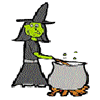Hubble, Bubble

Age 7 to 11 Challenge Level:

Winifred Wytsh bought a box each of jelly babies, milk jelly bears, yellow jelly bees and jelly belly beans. In how many different ways could she make a jolly jelly feast with 32 legs?Largest Number

Age 11 to 14 Challenge Level:

What is the largest number you can make using the three digits 2, 3 and 4 in any way you like, using any operations you like? You can only use each digit once.Calendar Calculations

Age 7 to 11 Challenge Level:

Try adding together the dates of all the days in one week. Now multiply the first date by 7 and add 21. Can you explain what happens?Eleven

Age 11 to 14 Challenge Level:

Replace each letter with a digit to make this addition correct.Abundant Numbers

Age 7 to 11 Challenge Level:

48 is called an abundant number because it is less than the sum of its factors (without itself). Can you find some more abundant numbers?The Pied Piper of Hamelin

Age 7 to 11 Challenge Level:

This problem is based on the story of the Pied Piper of Hamelin. Investigate the different numbers of people and rats there could have been if you know how many legs there are altogether!Arranging the Tables

Age 7 to 11 Challenge Level:

There are 44 people coming to a dinner party. There are 15 square tables that seat 4 people. Find a way to seat the 44 people using all 15 tables, with no empty places.Scoring with Dice

Age 7 to 11 Challenge Level:

I throw three dice and get 5, 3 and 2. Add the scores on the three dice. What do you get? Now multiply the scores. What do you notice?Cows and Sheep

Age 7 to 11 Challenge Level:

Use your logical reasoning to work out how many cows and how many sheep there are in each field.Number Differences

Age 7 to 11 Challenge Level:

Place the numbers from 1 to 9 in the squares below so that the difference between joined squares is odd. How many different ways can you do this?Score

Age 11 to 14 Challenge Level:

There are exactly 3 ways to add 4 odd numbers to get 10. Find all the ways of adding 8 odd numbers to get 20. To be sure of getting all the solutions you will need to be systematic. What about. . . .Making Sense of Positives and Negatives

Age 11 to 14

This article suggests some ways of making sense of calculations involving positive and negative numbers.Being Resilient - Primary Number

Age 5 to 11 Challenge Level:

Number problems at primary level that may require resilience.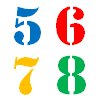How Old?

Age 7 to 11 Challenge Level:

Cherri, Saxon, Mel and Paul are friends. They are all different ages. Can you find out the age of each friend using the information?Escape from the Castle

Age 7 to 11 Challenge Level:

Skippy and Anna are locked in a room in a large castle. The key to that room, and all the other rooms, is a number. The numbers are locked away in a problem. Can you help them to get out?X Is 5 Squares

Age 7 to 11 Challenge Level:

Can you arrange 5 different digits (from 0 - 9) in the cross in the way described?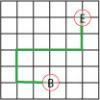Journeys in Numberland

Age 7 to 11 Challenge Level:

Tom and Ben visited Numberland. Use the maps to work out the number of points each of their routes scores.Take Three Numbers

Age 7 to 11 Challenge Level:

What happens when you add three numbers together? Will your answer be odd or even? How do you know?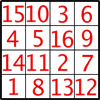Magic Constants

Age 7 to 11 Challenge Level:

In a Magic Square all the rows, columns and diagonals add to the 'Magic Constant'. How would you change the magic constant of this square?Countdown

Age 7 to 14 Challenge Level:

Here is a chance to play a version of the classic Countdown Game.Sums and Differences 1

Age 7 to 11 Challenge Level:

This challenge focuses on finding the sum and difference of pairs of two-digit numbers.Sums and Differences 2

Age 7 to 11 Challenge Level:

Find the sum and difference between a pair of two-digit numbers. Now find the sum and difference between the sum and difference! What happens?